# Segmenting an image: An Image Processing Introduction

Image segmentation is the process of partitioning an image into several segments or extracting a specific part or information of an image. For this article, I will discuss how we would be able to segment the image using two kinds of color segmentation: RGB and HSV, and also via image differentiation.

# Color segmentation

It is possible to segment images based on their color via RGB and HSV. Depending on which segmentation technique you will use, you will be able to extract a specific segment of the image as long as it is colored. Let’s use this colored balls for this one: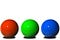RGB balls

## Via RGB color space

Let’s try extracting the blue ball via the RGB color space using the following code:

`fig, ax = plt.subplots(1, 3, figsize=(12,4))ax.imshow(balls[:,:,0], cmap=’Reds’)ax.set_title(‘Red’)ax.imshow(balls[:,:,1], cmap=’Greens’)ax.set_title(‘Green’)ax.imshow(balls[:,:,2], cmap=’Blues’)ax.set_title(‘Blue’);`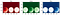Image showing each of the ball with most of the colors
`from skimage.filters import threshold_otsuballs_gray = rgb2gray(balls[:,:,0] < 100) & (balls[:,:,1] < 100) & (balls[:,:,2] > 100)imshow(balls_gray)thresh = threshold_otsu(balls_gray)balls_binary_otsu2 = balls_gray < threshimshow(balls_binary_otsu2)`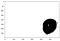Segmented blue ball in grayscale

After identifying the RGB color space values of the image, I used Otsu’s thresholding technique in order to fully segment the blue ball from the rest of the image based from the results above. The downside of using RGB color space is that if there are other colors that we need to segment, we need to find the correct RGB color channel values of that specific color in order to fully segment it.

## HSV color space

However, it is possible to extract those colors using HSV color space. We would be able to extract other colors much easier if we will use this technique.

`from skimage.color import rgb2hsvballs_hsv = rgb2hsv(balls)fig, ax = plt.subplots(1, 3, figsize=(12,4))ax.imshow(balls_hsv[:,:,0], cmap=’gray’)ax.set_title(‘Hue’)ax.imshow(balls_hsv[:,:,1], cmap=’gray’)ax.set_title(‘Saturation’)ax.imshow(balls_hsv[:,:,2], cmap=’gray’)ax.set_title(‘Value’);`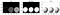HSV color channel values
`plt.imshow(balls_hsv[:,:,0],cmap=’hsv’)plt.colorbar()`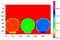Hue color channel
`lower_mask = balls_hsv[:,:,0] > 0.60upper_mask = balls_hsv[:,:,0] < 0.70saturation = balls_hsv[:,:,1] > 0.5mask = upper_mask * lower_mask * saturationred = balls[:,:,0]*maskgreen = balls[:,:,1]*maskblue = balls[:,:,2]*maskballs_masked = np.dstack((red,green,blue))imshow(balls_masked)`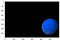Segmented blue ball

The implementation of segmenting the blue ball via HSV color space is a bit straightforward. Rather than using Otsu’s threshold, what we did here is get the lower mask and upper mask of the hue, and saturation value to show only the blue ball and mask all other parts of the image.

# Image differencing

Image differencing is one way of knowing what have changed in the image. What we do here is find the differences between the two images. This is a “good way” when solving “find the difference” puzzles. Let’s use these images for this one: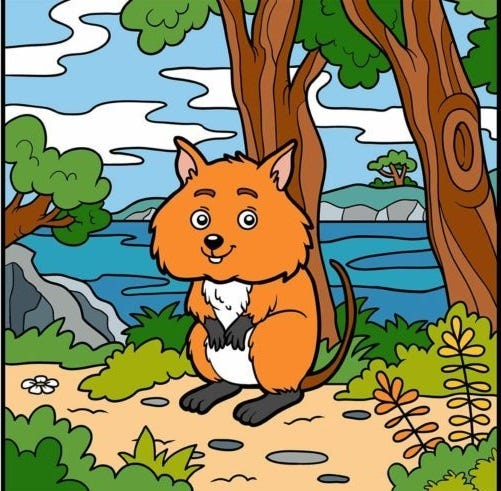Sample find the difference image

Some of you may have probably found the differences between the two image, but I want to “cheat” my way onto it:

`from skimage.color import rgb2grayimage1 = imread('spotdiff1.jpg')image2 = imread('spotdiff2.jpg')image1_gray = rgb2gray(image1)image2_gray = rgb2gray(image2)diff = image1_gray - image2_grayimshow(diff)`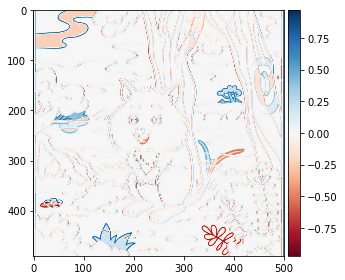Results of the difference between the two images

Using the implementation above, we were able to highlight the difference between the two images which are shown blue and red.

There you have it! That’s how we would be able to segment the images using color spaces and image differencing.

A developer by day, a programmer by night

## More from Cymon Marcaida

A developer by day, a programmer by night